# Motion Graphs

Distance-time graph is the plot of distance travelled by a body against time. So it will tell us about the journey made by a body and its speed. Below is an example of a distance-time graph, if it is a straight horizontal line then the body is stationary, and its speed is zero, if the line is diagonal then it is moving with a constant speed and if it is anything other than a straight line then the speed is varying.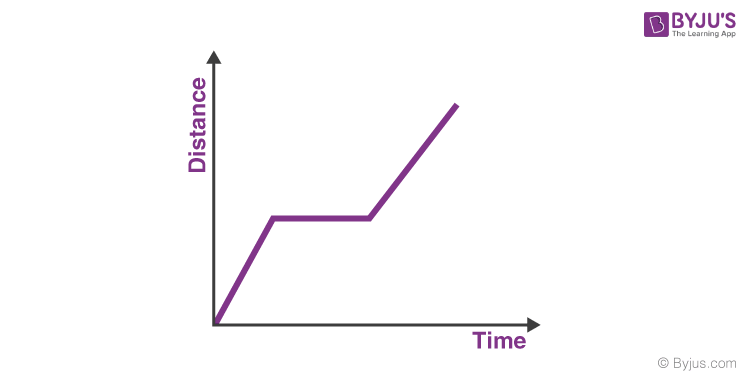## Distance-Time Graph for Uniform Motion

Let us now consider a distance-time graph in which the body is moving with uniform motion. A body is said to be in uniform motion when the body covers an equal distance in equal time intervals. Let’s consider a time interval of 1 second, If a body covers 10 meters in the first 1-second then it should cover 10 meters in every second from there on, this will indicate that the body is in uniform motion. Let’s draw a graph for uniform motion.

As in uniform motion, the distance-time graph would be a straight line, because the equal distance is covered in equal units of time.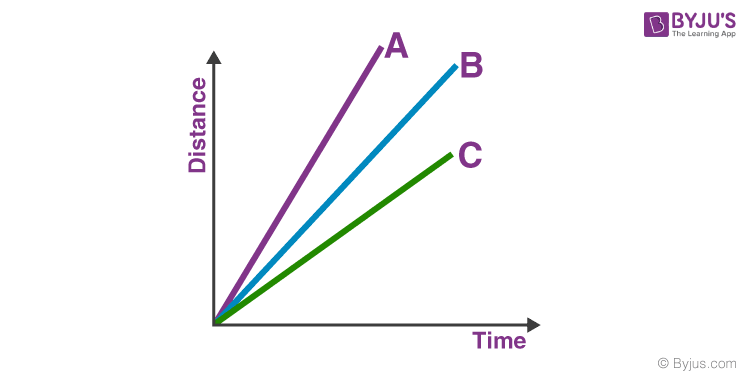You can see that there are three bodies A, B and C, all of them are in uniform motion then why do they have different slopes?

It is because the slope of a distance-time graph determines the speed of that body, so the steeper the slope greater will be the speed of the body. From the above graph, we can come to a conclusion that body A has the highest speed and body C has the least speed.

### Velocity-Time Graph

It is the graph of velocity against time; it shows us how the velocity changes with respect to time. The slope of a velocity-time graph determines its acceleration.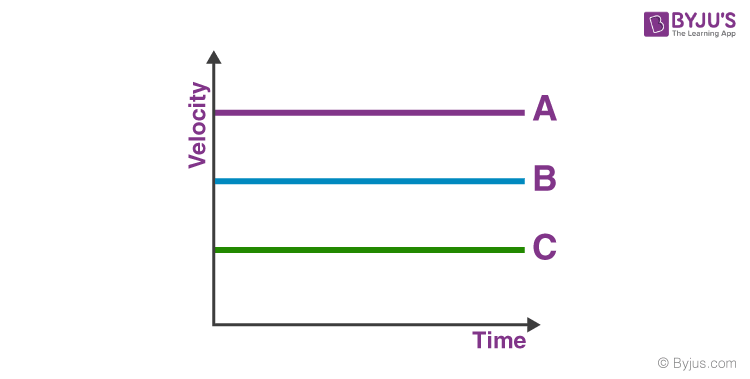Above is the velocity-time graph for three objects A, B, and C. A flat horizontal line in a velocity-time graph states that the body is moving at a constant velocity. If the straight line has a slope, then that indicates the body is changing its velocity at a constant rate, or it means that the body has constant acceleration.

#### Distance-Time Graph for Uniformly Accelerated Motion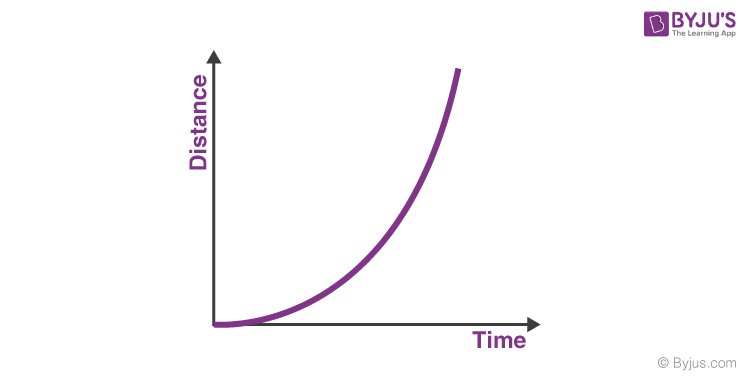The distance-time graph for a uniformly accelerated motion looks as shown in the graph above, consider how the distance is changing exponentially indicating that the velocity is changing at a constant rate or there is constant acceleration. But in the velocity-time graph as velocity changes at a constant rate with respect to time in uniformly accelerating motion, the graph would be a straight line with its slope indicating the amount of acceleration.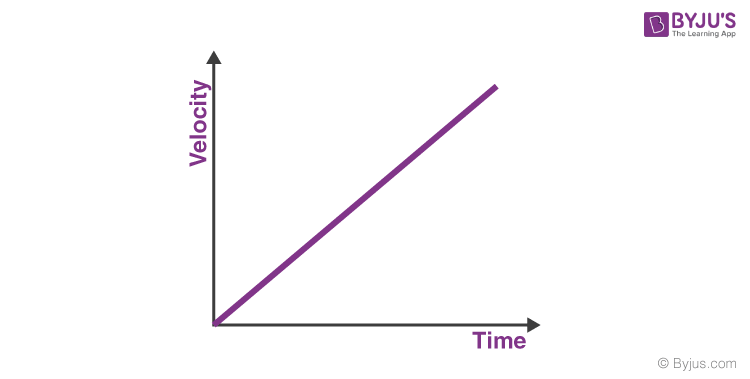## The complete quiz of the chapter Motion Class 9## Top 10 NTSE Important Questions on Motion Class 9